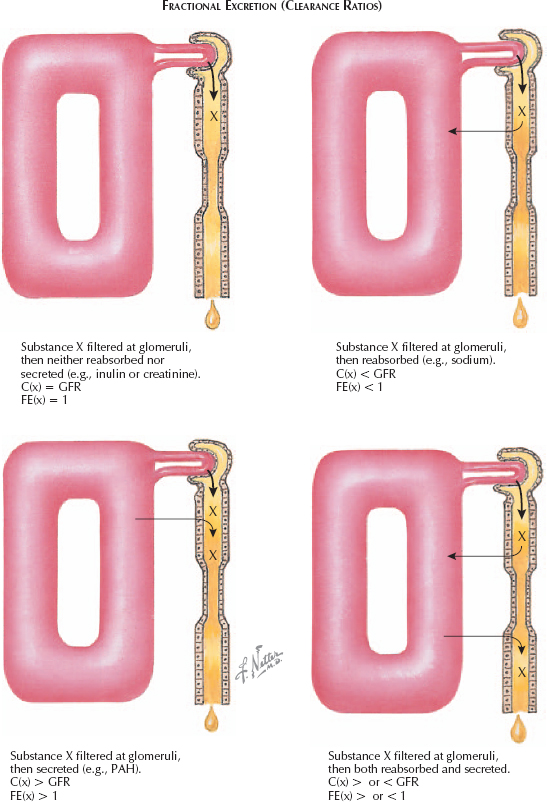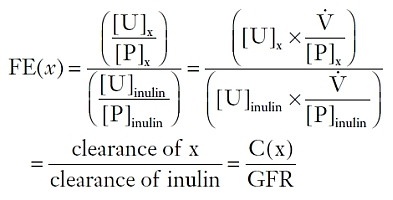# Secretion and Reabsorption: Fractional Excretion (Clearance Ratios)

### SECRETION AND REABSORPTION(Continued)

#### QUANTIFYING REABSORPTION AND SECRETION

One means of assessing the secretion or reabsorption profile of a particular solute is to examine its changing concentration along the length of the nephron. As filtrate enters Bowman’s space, each solute x has an initial tubular concentration, [T]x. For substances that freely cross the glomerular capillaries, the initial [T]x equals the plasma concentration, [P]x. As filtrate progresses through the nephron, however, [T]x/[P]x changes. If it exceeds one, the tubular concentration of x has increased, suggesting either secretion of x or reabsorption of water. If [T]x/[P]x remains equal to one, there has been either no secretion or reabsorption, or there has been equal reabsorption of x and water. Thus in all instances one must control for the reabsorption of water. Because inulin is, as previously described, a compound that is filtered but neither secreted nor reabsorbed, one can use its concentration to infer water reabsorption. If [T]inulin doubles relative to [P]inulin, then half of the water has been reabsorbed.

In Bowman’s space, ([T]x/[P]x) = ([T]inulin/[P]inulin) = 1. If, at some later point in the nephron, ([T]x/[P]x)/ ([T]inulin/[P]inulin) = 1/2, it suggests that half of the water, as well as half of solute x, have been reabsorbed. If none of solute x had been reabsorbed, the quotient would be equal to 1, since removing half of the filtered water would have doubled the tubular concentration of x relative to plasma, just as for inulin.

One can assess the overall reabsorption and secretion pattern of any given solute by examining ([U]x/[P]x) / ([U]inulin/[P]inulin), a ratio known as the fractional excretion (FE) of x. For those substances that are not freely filtered at the glomerulus, such as ions partially bound to plasma proteins, the plasma concentration should be multiplied by the free fraction.If FE(x) equals 0.01, then 99% of the filtered x has been reabsorbed. In contrast, if FE(x) is greater than 1, then there has been net secretion of x. FE(x) can also be viewed as a “clearance ratio,” which may be easier to conceptualize:Clearance ratios greater than one suggest that X undergoes net secretion, whereas values less than one suggest that X undergoes net reabsorption.

In clinical practice, creatinine is used in lieu of inulin to calculate fractional excretion values (or clearance ratios) because it is an endogenous chemical that is generally at a steadystate concentration in plasma.

< div class='tao-gold-member'>

Only gold members can continue reading. Log In or Register to continue

Jul 4, 2016 | Posted by in UROLOGY | Comments Off on Secretion and Reabsorption: Fractional Excretion (Clearance Ratios)
Premium Wordpress Themes by UFO Themes